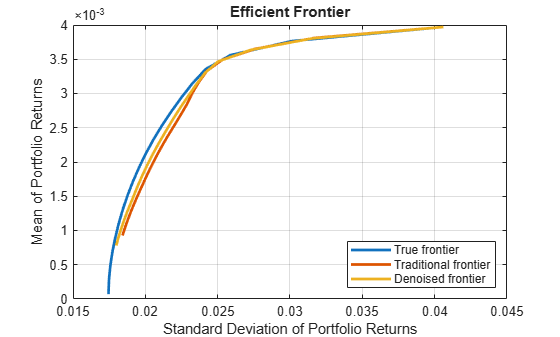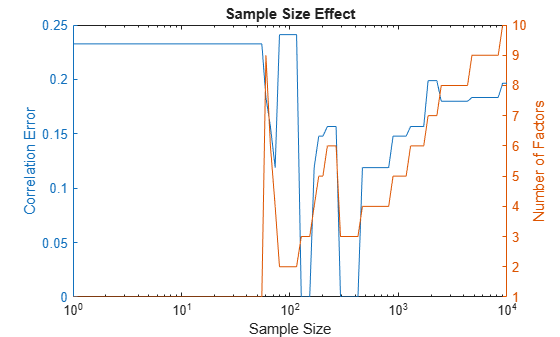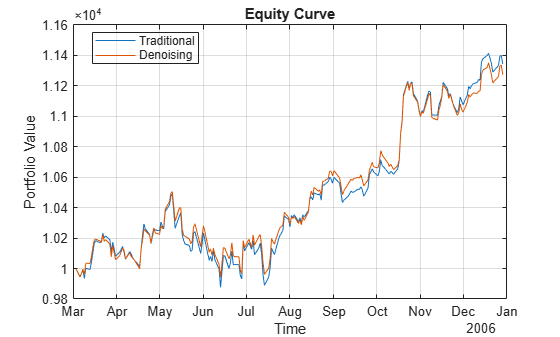# covarianceDenoising

Estimate covariance matrix using denoising

Since R2023a

## Syntax

``SigmaHat = covarianceDenoising(AssetReturns)``
``SigmaHat = covarianceDenoising(Sigma,SampleSize)``
``[SigmaHat,numSignalEig] = covarianceDenoising(___)``

## Description

example

````SigmaHat = covarianceDenoising(AssetReturns)` returns a covariance estimate that uses random matrix theory to denoise the empirical covariance matrix. For more information, see Covariance Denoising and Denoising Algorithm.In addition, you can use `covarianceShrinkage` to compute an estimate of covariance matrix using shrinkage estimation. For information on which covariance estimation method to choose, see Comparison of Methods for Covariance Estimation.```

example

````SigmaHat = covarianceDenoising(Sigma,SampleSize)` returns a covariance estimate from an initial covariance matrix estimate (`Sigma`) and the sample size used to estimate the initial covariance (`sampleSize`).```

example

````[SigmaHat,numSignalEig] = covarianceDenoising(___)` returns a covariance estimate and the number of eigenvalues that are associated with signal in combination with either of the input argument combinations in the previous syntaxes.```

## Examples

collapse all

This example shows how to use covariance denoising to reduce noise and enhance the signal of the empirical covariance matrix. In mean-variance portfolio optimization, a noisy estimate of the covariance estimate results in unstable solutions that cause high turnover and transaction costs. Ideally, to decrease the estimation error, it is desirable to increase the sample size. Yet, there are cases where this is not possible. In extreme cases in which the number of assets is larger than the number of observations, the traditional covariance matrix results in a singular matrix. Working with a nearly singular or an ill-conditioned covariance matrix magnifies the impact of estimation errors.

Compute a portfolio efficient frontier using different covariance estimates with the same sample data.

```% Load portfolio data with 225 assets load port5.mat covariance = corr2cov(stdDev_return,Correlation); % Generate a sample with 200 observations rng('default') nScen = 200; retSeries = portsim(mean_return',covariance,nScen);```

Compute the traditional and denoised covariance estimates. Use `covarianceDenoising` to create a covariance estimate.

```Sigma = cov(retSeries); denoisedSigma = covarianceDenoising(retSeries);```

Compute the condition number of both covariance estimates. The denoised covariance `denoisedSigma` has a lower condition number than the traditional covariance estimate `Sigma`.` `

```conditionNum = [cond(Sigma); cond(denoisedSigma)]; condNumT = table(conditionNum,'RowNames',{'Sigma','SigmaHat'})```
```condNumT=2×1 table conditionNum ____________ Sigma 4.091e+18 SigmaHat 1046.8 ```

Use `Portfolio` to construct `Portfolio` objects that use the different `AssetCovar` values. Then use `setDefaultConstraints` to set the portfolio constraints with nonnegative weights that sum to 1 for the three portfolios: the true mean with the true covariance, the traditional covariance estimate, and the denoised estimate.

```% Create a Portfolio object with the true parameters p = Portfolio(AssetMean=mean_return,AssetCovar=covariance); p = setDefaultConstraints(p); % Create a Portfolio object with true mean and traditional covariance estimate pTraditional = Portfolio(AssetMean=mean_return,AssetCovar=Sigma); pTraditional = setDefaultConstraints(pTraditional); % Create a Portfolio object with true mean and denoised covariance pDenoised = Portfolio(AssetMean=mean_return,AssetCovar=denoisedSigma); pDenoised = setDefaultConstraints(pDenoised);```

Use `estimateFrontier` to estimate the efficient frontier for each of the `Portfolio` objects.

```% Number of portfolios on the efficient frontier nPort = 20; % True efficient portfolios w = estimateFrontier(p,nPort); % Traditional covariance efficient portfolios wTraditional = estimateFrontier(pTraditional,nPort); % Denoised covariance efficient portfolios wDenoised = estimateFrontier(pDenoised,nPort);```

Use `plotFrontier` to plot the frontier obtained from the different weights using the true parameter values.

```figure plotFrontier(p,w) hold on plotFrontier(p,wTraditional) plotFrontier(p,wDenoised) legend('True frontier','Traditional frontier','Denoised frontier', ... Location='southeast'); hold off```The efficient frontier obtained by denoising is closer to the true frontier than the frontier obtained using the traditional covariance estimate. This improvement shows the robustness of the denoised covariance estimate.

This example shows the effect of the sample size in `covarianceDenoising` to compute a denoised version of an initial covariance estimate. The `covarianceDenoising` function has two different syntaxes. This example focuses on the syntax with two inputs: a covariance estimate and the size of the sample that you use to compute the initial covariance estimate. This example demonstrates an empirical study of the effect of the sample size on the acuracy of the denoised estimate. The goal of this example is to provide insight for the choice of the sample size when this information is not available. This scenario can happen if the initial covariance estimate is stored but the information of the sample used to compute the estimate is not available.

Create Random Sample

Create a random sample using `mvnrnd`.

```% Load data load('port5.mat','mean_return','Correlation') % Select the number of assets numObservations = 300; numAssets = 100; mu = mean_return(1:numAssets); C = Correlation(1:numAssets,1:numAssets); % Generate a random sample rng('default') randSample = mvnrnd(mu,C,numObservations);```

Use `corr` to compute the standard correlation estimate of the sample. This example works with the correlation matrix to remove the effect of the variance. This is useful when comparing the effect of the sample size in the denoised covariance estimate.

`stdCorr = corr(randSample);`

Due to numerical errors, `stdCorr` is not symmetric. Modify `stdCorr` to make it symmetric.

```% Compute maximum absolute difference of matrix against its % transpose max(abs(stdCorr-stdCorr'),[],'all')```
```ans = 1.1102e-16 ```
```% Force correlation matrix to be symmetric stdCorr = (stdCorr+stdCorr')/2;```

Use `covarianceDenoising` to compute the denoised covariance estimate from `Sigma` using the correct sample size.

```denoisedCorr = covarianceDenoising(stdCorr,numObservations); denoisedCorr = (denoisedCorr+denoisedCorr')/2;```

Compute Denoised Covariance for Different Sample Sizes

Compare the maximum correlation difference between the denoised covariance with the correct sample size and other sample sizes. Also, you compute the number of factors.

```% Sample sizes to try numSizes = 100; sampleSizes = ceil(logspace(0,4,numSizes)); % Compute denoised correlation and store the maximum correlation % mismatch correlations = cell(numSizes); numFactors = zeros(numSizes,1); maxDiff = zeros(numSizes,1); for i = 1:numSizes [correlations{i},numFactors(i)] = covarianceDenoising(stdCorr,... sampleSizes(i)); maxDiff(i) = norm(correlations{i}(:)-denoisedCorr(:),inf); end```

Plot the maximum error in the correlations as well as the number of factors identified for each sample size.

```figure; % Plot correlation error yyaxis left semilogx(sampleSizes,maxDiff) hold on ylabel("Correlation Error"); % Plot number of factors yyaxis right semilogx(sampleSizes,numFactors) ylabel("Number of Factors"); % Title, labels, and legends title("Sample Size Effect") xlabel("Sample Size"); hold off```Note that when the sample size is close to the number of assets, the behavior of both the correlation error and the number of factors is erratic. Here, when the sample size is between 55 and 292, the number of factors and the correlation error jump up and down. Before 55 "observations" the number of factors is 1 because the denoising method doesn't have enough information to trust that what is being observed is signal and not noise; only the largest eigenvalue is associated with signal. Usually the largest eigenvalue is linked to the market. Meanwhile, after 292 "observations" the number of eigenvalues not associated with noise monotonically increases. This behavior happens because denoising assumes that the sample is less noisy with more observations, so identifying signal from noise is easier.

If the sample size of the initial covariance estimate is not known, it is better to choose a sample size that is far from the number of assets. For cases with less trust in the initial covariance estimate, the sample size should be smaller, below 55 in this example. If you trust the initial covariance estimate, then the sample size should be larger, above 292 in this example. The degree of trust in the initial covariance matrix can be adjusted by increasing the sample size.

This example compares a minimum variance investment strategy using the traditional covariance estimate with a minimum variance strategy using covariance denoising.

```% Read a table of daily adjusted close prices for 2006 DJIA stocks. T = readtable('dowPortfolio.xlsx'); % Convert the table to a timetable. pricesTT = table2timetable(T,'RowTimes','Dates'); numAssets = size(pricesTT.Variables, 2);```

Use the first 42 days of the `dowPortfolio.xlsx` data set to initialize the backtest strategies. The backtest is then run over the remaining data.

`warmupPeriod = 42;`

Compute Initial Weights

Use the `traditionalStrat` and `denoisedStrat` functions in Local Functions to compute the weights.

```% Specify no current weights (100% cash position). w0 = zeros(1,numAssets); % Specify warm-up partition of data set timetable. warmupTT = pricesTT(1:warmupPeriod,:); % Compute the initial portfolio weights for each strategy. traditional_initial = traditionalStrat(w0,warmupTT); shrunk_initial = denoisedStrat(w0,warmupTT);```

Create Backtest Strategies

Create `Traditional` and `Denoising` backtest strategy objects using `backtestStrategy`.

```% Rebalance approximately every month. rebalFreq = 21; % Set the rolling lookback window to be at least 2 months and at % most 6 months. lookback = [42 126]; % Use a fixed transaction cost (buy and sell costs are both 0.5% % of amount traded). transactionsFixed = 0.005; % Specify the strategy objects. strat1 = backtestStrategy('Traditional', @traditionalStrat, ... RebalanceFrequency=rebalFreq, ... LookbackWindow=lookback, ... TransactionCosts=transactionsFixed, ... InitialWeights=traditional_initial); strat2 = backtestStrategy('Denoising', @denoisedStrat, ... RebalanceFrequency=rebalFreq, ... LookbackWindow=lookback, ... TransactionCosts=transactionsFixed, ... InitialWeights=shrunk_initial); % Aggregate the two strategy objects into an array. strategies = [strat1, strat2];```

Create a `backtestEngine` object then use `runBacktest` to run the backtest.

```% Create the backtesting engine object. backtester = backtestEngine(strategies); % Run the backtest. backtester = runBacktest(backtester,pricesTT,'Start',warmupPeriod); % Generate summary table of the performance of the strategies. summary(backtester)```
```ans=9×2 table Traditional Denoising ___________ __________ TotalReturn 0.13431 0.12745 SharpeRatio 0.10807 0.10719 Volatility 0.0057472 0.0055101 AverageTurnover 0.0098378 0.0074213 MaxTurnover 0.36237 0.2791 AverageReturn 0.0006196 0.00058922 MaxDrawdown 0.058469 0.053624 AverageBuyCost 0.51636 0.38982 AverageSellCost 0.51636 0.38982 ```

Compare Performance of Strategies

Use `equityCurve` to plot the equity curve to compare the performance of both strategies.

`equityCurve(backtester)`The maximum and average turnover are decreased using covariance denoising. Also, the covariance denoising strategy results in a decrease of buy and sell costs. In this example, not only is the turnover decreased, but also the volatility and the maximum drawdown are decreased. Therefore, in this example the denoised covariance produces more robust weights.

Local Functions

```function new_weights = traditionalStrat(~, pricesTT) % Function for minimum variance portfolio using traditional covariance estimate. % Compute the returns from the prices timetable. assetReturns = tick2ret(pricesTT); mu = mean(assetReturns.Variables); Sigma = cov(assetReturns.Variables,"omitrows"); % Create the portfolio problem. p = Portfolio(AssetMean=mu,AssetCovar=Sigma); % Specify long-only fully invested contraints. p = setDefaultConstraints(p); % Compute the minimum variance portfolio. new_weights = estimateFrontierLimits(p,'min'); end function new_weights = denoisedStrat(~, pricesTT) % Function for minimum variance portfolio using covariance denoising. % Compute the returns from the prices timetable. assetReturns = tick2ret(pricesTT); mu = mean(assetReturns.Variables); Sigma = covarianceDenoising(assetReturns.Variables); % Create the portfolio problem. p = Portfolio(AssetMean=mu,AssetCovar=Sigma); % Specify long-only fully invested contraints. p = setDefaultConstraints(p); % Compute the minimum variance portfolio. new_weights = estimateFrontierLimits(p,'min'); end```

## Input Arguments

collapse all

Asset returns, specified as a `NumObservations`-by-`NumAssets` matrix, table, or timetable.

Note

All rows (observations) with one or more `NaN` values are removed before computing the covariance estimate.

Data Types: `double` | `table` | `timetable`

Covariance matrix estimate, specified as a `NumAssets`-by-`NumAssets` covariance estimate matrix.

Data Types: `double`

Sample size to compute the initial covariance estimate `Sigma`, specified as a scalar numeric value.

Data Types: `double`

## Output Arguments

collapse all

Estimated covariance matrix, returned as a `NumAssets`-by-`NumAssets` matrix.

Number of eigenvalues associated with signal, returned as a scalar numeric.

collapse all

### Covariance Denoising

The goal of covariance denoising is to reduce the noise and enhance the signal of the empirical covariance matrix.

Covariance denoising is a common method that is used to reduce the effect of noise in the covariance approximation. Covariance denoising reduces the ill-conditioning of the traditional covariance estimate by differentiating the eigenvalues associated with noise from the eigenvalues associated with signal. Random matrix theory is used in covariance denoising to pull only the eigenvalues that are associated with noise to a common mean.

An intuitive example is when the number of variables is larger than the number of observations. In this case, the result is a noninvertible covariance matrix. Furthermore, because covariance estimation is performed using observations from random data, the estimator contains a certain amount of noise. This noise usually results in ill-conditioned covariance estimates. Working with an ill-conditioned matrix magnifies the impact of estimation errors.

Unlike shrinkage methods, denoising does not require information from the full sample. In covariance denoising using `covarianceDenoising` you only need to know the number of scenarios that were used to estimate the covariance matrix.

## Algorithms

The `covarianceDenoising` function shrinks only the part of the covariance that corresponds with noise as follows:

1. Compute the correlation matrix C associated with the traditional covariance estimate Σ.

2. Compute the eigendecomposition of C = VΛ VT.

3. Estimate the empirical distribution of the eigenvalues using kernel density estimation with `fitdist(x,'Kernal')`. For more information, see `fitdist`.

4. Fit the Marchenko-Pastur distribution to the empirical distribution by minimizing the mean squared error (MSE) between the empirical probability density function (pdf) and the fitted Marchenko-Pastur pdf. This gives the theoretical bounds λ+ and λ- on the eigenvalues associated with noise.

5. Let $\stackrel{-}{\lambda }$ be the average of the eigenvalues smaller than λ+. Set all eigenvalues smaller than λ+ to $\stackrel{-}{\lambda }$. These are the eigenvalues associated with noise.

6. Compute the denoised version of the correlation matrix $\stackrel{^}{C}=V\stackrel{-}{\Lambda }{V}^{T}$ and rescale $\stackrel{^}{C}$ so that the main diagonal only has ones. $\stackrel{^}{C}$ is a correlation matrix.

7. Compute the denoised covariance estimate $\stackrel{^}{\Sigma }$ from $\stackrel{^}{C}$.

 Lòpez de Prado, M. Machine Learning for Asset Managers (Elements in Quantitative Finance). Cambridge University Press, 2020.